## How do I solve completely this differential equati...

How do I solve completely the diferential equation and also speed up the compilation of  the time is over 3000sec:

eq1 := 2*m*(E + 8*Pi*epsilon/r)*f(r, t)/h^2 + R*diff(f(r, t), r \$ 2)/r - diff(f(r, t), t \$ 2)/(a^2*c^2) = 0;

iv1 := f(r, 0) = 0, f(R, t) = 0, D(f)(0, 0) = R;

Sol := pdsolve([eq1, iv1]);

Where f(r,t) is the function of variable r and t  in spherical coordinate and m, E, h, R, rb, a, and c are constants.

I also want to find the exact value of f(r,t) with the condition f(rb, 0) = 0; and diff(f(rb,t),t)=a*c for the value t=0 and if is possible the pulsation of the sinusoidal solution of f(r,t). [the solution is a combination of AiryAi ; AiryBi and sinusoidal sin(a*c*sqrt(-2*E*m - _c)*t/h)]. I didn't find the value of _c for the 2 additional condition above.

The issue is the period of time between 2 consecutive zero of the f(r,t)=0

tks

## Can we create more graphs with this function Sequ...

I get the degree sequence of a graph, I want to create more graphs, but maple seems to only get the only graph.

Is there a good way to get more, a foolish dream, can we get all the graphs that satisfy this degree sequence condition?

with(GraphTheory):
L:=[7\$1..22,6]:
IsGraphicSequence(L):
G := SequenceGraph(L):
DrawGraph(%)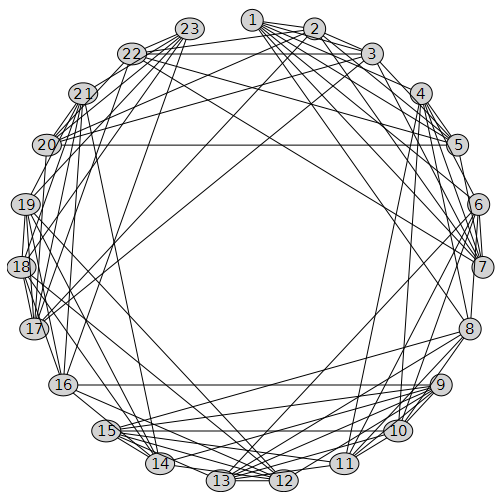## Distance between vertex and edge ...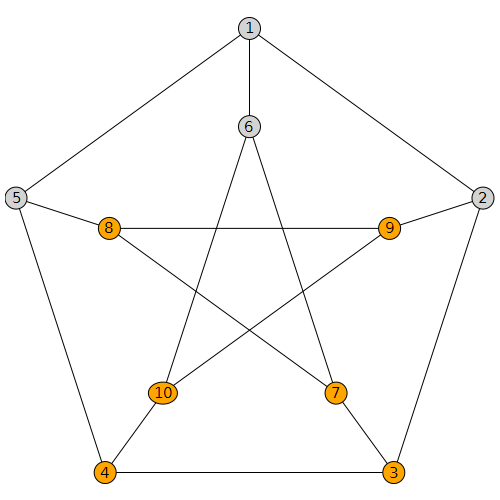What I mean by shortest distance between vertex and edge is

In the above petersen graph consider the vertex say 1 it's distance to

(1,6) will be 0 as 1 is part of the same edge

((1,2) will be 0 again

(1,5) it will zero again

(5,8) will be 1 that is (1,5)-(5,8)

(8,9) it will be 2 that is (1,5)-(5,8)-(8,9)

(5,4) it will be 1 that is (1,5)-(5,4)

(9,10) it will be 2 (1,5)-(5,4)-(9,10)

Etc for all edges the graph from a given vertex v given a Graph G

The order in which the edges are chosen to find the distances should be maintained so that each index in the list correspond to distance of that vertex from the same edge in different vertex lists in a order

Kind help I apologize to take your time

In output I need a list say T=[0, 0,1,2,..]

## Form a table output from a list data and to export...

Data given

List Given

L:=[A,B,A,C,A,B,D,E,A,F,G,H,H,G,I,P,Q,W,A]

I want the output to be a frequency table

Frequency says number of times each Data occurs in the list

I want output in the form of a table

Data need not be always A,B,C,D etc it can be any text.

 Data Frequency A 5 B 2 C 1 D 1 E 1 F 1 G 2 H 2 I 1 P 1 Q 1 W 1

I want to export this outputed table to word or Excel

## Problem with animation toolbar in aple 2021...

Anyone have a problem with non-appearance of the animation toolbar in Maple 2021????

Whilst coming up with a response for the problem here

https://www.mapleprimes.com/questions/231862-Have-You-Ever-Heard-Of-Vector-Asterisks-

I found an issue with the non-appearance of the animation toolbar in Maple 2021. This is rather difficult to illustrate without the use of screenshots, for which I apologise.

Normally(?) I would just select a plot and the animation toolbar appears "as if by magic"

First screen shot is using Maple 2020, The blue highlighting rectangle around the plot was visible when I initiated the snip, but disappeared when the snipping tool activated. However this shows that the animation toolbar is available (and works)I do exactly the same thing in  Maple 2021 and I can't make the animation toolbar appear - see below. Aagain the plot wa highlighted when I initiated the snip but the highlighting disappeared when the snipping tool activated. Now there is no sign of the animation toolbarIt is still possible to do very basic animation in Maple 2021 by clicking on the plot and using the context menu - but this is very basic

The code used in the above plots is given supplied below

```  restart;
kernelopts(version);
plots:-display
( [ seq
( plot
( Vector([1, 3, 4, 6]),
Vector([8, 6, 2, 5]),
style = point,
symbol = j,
symbolsize = 40,
color = blue
),
j in [ asterisk, box, circle, cross, diagonalcross,
diamond, point, solidbox, solidcircle, soliddiamond
]
)
],
insequence = true
);
```

Is it just me?

## Why does function plot correctly but returns wrong...

I have defined a function pp(x).  It plots correctly

but pp(2020); should return 410, and it returns 98000. Maybe I am doing something stupid, but I just can't figure out what!

a := 0.340699639252428*10^13;
b := -0.118229737138742*10^11;
c := 0.175816922773262*10^8;
d := -14523.7138112711;
e := 7.19782673654200;
f := -0.00214008825847444;
g := 0.353463615941623*10^(-6);

h := -0.250170509163729*10^(-10);

pp := x -> a + b*x + c*x^2 + d*x^3 + e*x^4 + f*x^5 + g*x^6 + h*x^7;

plot([pp(x)], x = 1960 .. 2100, gridlines = true, size = [900, 600])## how do I handle rho:=x[n]/h in this code?...

Good day, all. I hope we are staying safe.

Please I need help with the regard to the following codes.

The major issue is with rho:=x[n]/h. The rho needs to change values as x[n] changes in the computation. This I think I have gotten wrong. Your professional modifications or/and corrections to the code would be appreciated.

Thank you and kind regards.

```restart;
Digits:=20:
#assume(alpha>0,alpha < 1):
f:=proc(n)
-y[n]-x[n]+(x[n])^2+(2*(x[n])^(2-alpha))/GAMMA(3-alpha)+((x[n])^(1-alpha))/GAMMA(2-alpha):
end proc:

e2:=y[n+2] = y[n]+(1/2)*((alpha^2-alpha)*rho^2+(2*alpha^2-2)*rho+(4/3)*alpha^2-(2/3)*alpha)*(rho*h)^alpha*GAMMA(2-alpha)*f(n)/rho-alpha*GAMMA(2-alpha)*((-1+alpha)*rho^2+(2*alpha-2)*rho+(4/3)*alpha-8/3)*(h*(rho+1))^alpha*f(n+1)/(rho+1)+(1/2)*((alpha^2-alpha)*rho^2+2*(-1+alpha)^2*rho+(4/3)*alpha^2-(14/3)*alpha+4)*(h*(rho+2))^alpha*f(n+2)*GAMMA(2-alpha)/(rho+2):
e1:=y[n+1] = y[n]+(1/4)*((alpha^2-alpha)*rho^2+(alpha^2+3*alpha-4)*rho+(1/3)*alpha^2+(4/3)*alpha)*GAMMA(2-alpha)*f(n)/((rho*h)^(-alpha)*rho)-(1/2)*GAMMA(2-alpha)*((alpha^2-alpha)*rho^2+(alpha^2+alpha-2)*rho+(1/3)*alpha^2+(1/3)*alpha-2)*f(n+1)/((h*(rho+1))^(-alpha)*(rho+1))+(1/4)*((-1+alpha)*rho^2+(-1+alpha)*rho+(1/3)*alpha-2/3)*alpha*GAMMA(2-alpha)*f(n+2)/((h*(rho+2))^(-alpha)*(rho+2)):

alpha:=0.25:
inx:=0:
iny:=0:
#x:=0:
h:=1/20:
N:=solve(h*p = 1, p):
n:=0:
c:=1:
rho:=x[n]/h:
err := Vector(round(N)):
exy_lst := Vector(round(N)):

for j from 0 to 2 do
t[j]:=inx+j*h:
end do:
vars:=y[n+1],y[n+2]:

step := [seq](eval(x, x=c*h), c=1..N):

printf("%4s%15s%15s%16s\n",
"h","Num.y","Ex.y","Error y");
st := time():
for k from 1 to N/2 do

par1:=x[n]=t,x[n+1]=t,x[n+2]=t:
par2:=y[n]=iny:
res:=eval(<vars>, fsolve(eval({e||(1..2)},[par1,par2]), {vars}));

for i from 1 to 2 do
exy:=eval(-c*h+(c*h)^2):
printf("%5.3f%17.9f%15.9f%15.5g\n",
h*c,res[i],exy,abs(res[i]-exy)):

err[c] := abs(evalf(res[i]-exy)):
exy_lst[c] := exy:
numerical_y1[c] := res[i]:
c:=c+1:
rho:=rho+1:
end do:
iny:=res:
inx:=t:
for j from 0 to 2 do
t[j]:=inx + j*h:
end do:
end do:
v:=time() - st;
printf("Maximum error is %.13g\n", max(err));

numerical_array_y1 := [seq(numerical_y1[i], i = 1 .. N)]:

time_t := [seq](step[i], i = 1 .. N):

with(plots):
numerical_plot_y1 := plot(time_t, numerical_array_y1, style = [point], symbol = [asterisk],
color = [blue,blue],symbolsize = 15, title="y numerical",legend = ["Numerical"]);
exact_plot_y1 := plot(time_t, exy_lst, style = [line], symbol = [box],
color = [red,red], symbolsize = 10, title="y exact",legend = ["Exact"]);

display({numerical_plot_y1, exact_plot_y1}, title = "Exact and Numerical Solution of Example 1 ");```

## how can i solve operate differential equation...

how can i solve this operate differential equation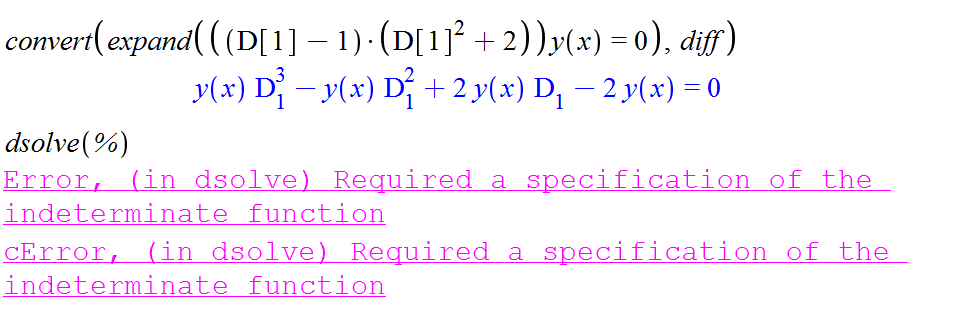convert(expand((D - 1)*(D^2 + 2)*y(x) = 0), diff);
dsolve(%)

## Does table have a predefined function to get indic...

Consider an easy example of a table.

`myTable:=table(["a"=1,"b"=-1,"c"=1]);`

We can give an index to it and get its corresponding entry if exists.

`myTable["b"];`

We can also get the set of all indices, or the set of all entries. But what about receiving the index or set of indices with a specific entry. for example asking what indices have the entry `1`?

Of course I can define a search procedure myself, but I thought there might be an efficient way which is already implemented as a function/method on tables.

## Numbering of constants...

Good afternoon. How do I disable the numbering of _Z constants? Because
of this, you can't use the procedure in a loop
Is it possible to disable numbering or do something else?

 >(1)
 >>(2)
 >(3)

## pdsolve exception (in GAMMA) numeric exception: di...

This is new exception generated by Maple pdsolve in 2021. Different from the last post I gave on pdsolve. So I thought it will be better to keep them separate since the causes are different.

```interface(version)
restart;
pde :=  diff(w(x,y,z),x)+(a1*x^n1*y+b1*x^m1)*diff(w(x,y,z),y)+(a2*x^n2*y+b2*x^m1)*diff(w(x,y,z),z)= 0;
pdsolve(pde,w(x,y,z));

#another example

restart;
local gamma:
pde := diff(w(x,y,z),x)+(a1*x^n1*y+ b1*x^m1)*diff(w(x,y,z),y)+(a2*x^n2*y+b2*x^m2)*diff(w(x,y,z),z)=c2*x^k2*y+c1*x^k1*z;
pdsolve(pde,w(x,y,z));

```

Error, (in GAMMA) numeric exception: division by zero

The same PDE works in 2020.2. The answer it gives is large so will not show it all below.

Screen shots

Maple 2021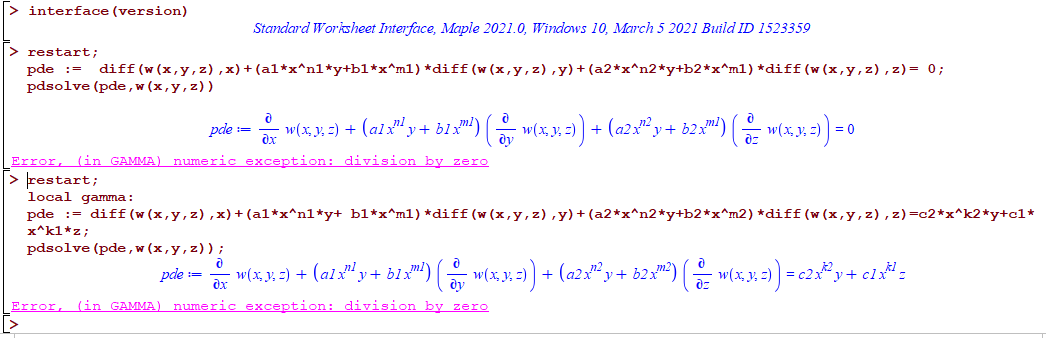Maple 2020.2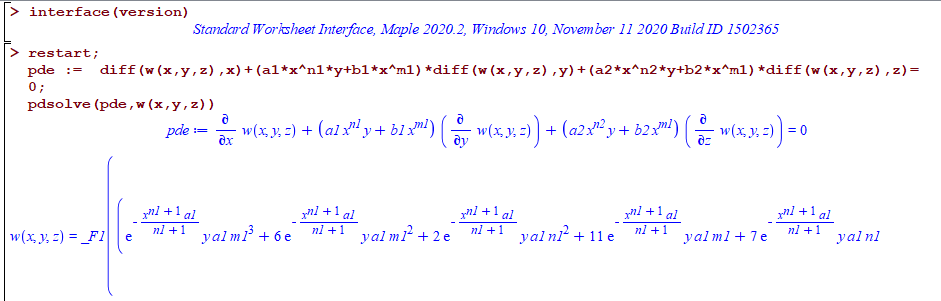All on windows 10.

Do other see the same error? What causes it?

## seq - numelems and declaring a local variable in ...## inert % does not obey order of operations...

4 %+ 3 %* 2;
%*(%+(4, 3), 2)

= (4+3)*2

It makes using % very difficult in complex expressions because one has to constantly use a ton of paranethesis just to get things to work out.

1 + 4%^3  %* 2 %- 6 %/ 4;

1 + ((((4 %^ 3) %* 2) %- 6) %/ 4)

= 1 + (4^3*2 - 6)/4

## pdsolve exception int/gbinthm/structure INVALID s...

I noticed number of pde's now fail in Maple 2021 with the error

int/gbinthm/structure INVALID subscript selector

but they do not fail in Maple 2020.2.

Here are few   examples

```restart;
pde :=a*x^n*diff(w(x,y),x) + n*x^m*y*diff(w(x,y),y) =s*x^p*y^q+d;
pdsolve(pde,w(x,y));

restart;
pde :=a*x^n*diff(w(x,y),x) + n*x^m*y*diff(w(x,y),y)=c*x^k*y^s+d;
pdsolve(pde,w(x,y))

restart;
pde :=a*x^n*diff(w(x,y),x)+b*x^m*y*diff(w(x,y),y) =  (c*x^k*y^s + d)*w(x,y);
pdsolve(pde,w(x,y))

restart;
pde :=  a*diff(w(x,y),x)+ y*diff(w(x,y),y) = b*w(x,y)+ c*x^n*y^m;
pdsolve(pde,w(x,y))

#etc..```

Error, (in int/gbinthm/structure) invalid subscript selector

While in Maple 2020.2 they all work. Screen shot

Maple 2021